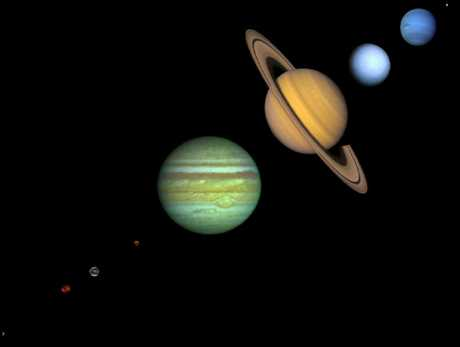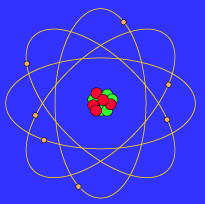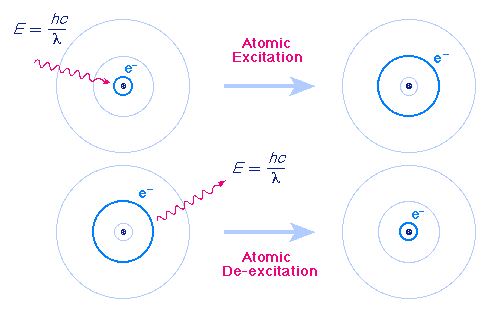The BohrModel

The most important properties of atomic and molecular structure may be exemplified using a simplified picture of an atom that is called the Bohr Model. This model was proposed by Niels Bohr in 1915; it is not completely correct, but it has many features that are approximately correct and it is sufficient for much of our discussion. The correct theory of the atom is called quantum mechanics; the Bohr Model is an approximation to quantum mechanics that has the virtue of being much simpler. (Here is a more realistic discussion of what atomic orbitals look like in quantum mechanics.)

## A Planetary Model of the AtomThe Bohr atom
The Bohr Model is probably familar as the "planetary model" of the atom illustrated in the adjacent figure that, for example, is used as a symbol for atomic energy (a bit of a misnomer, since the energy in "atomic energy" is actually the energy of the nucleus, rather than the entire atom). In the Bohr Model the neutrons and protons (symbolized by red and blue balls in the adjacent image) occupy a dense central region called the nucleus, and the electrons orbit the nucleus much like planets orbiting the Sun (but the orbits are not confined to a plane as is approximately true in the Solar System). The adjacent image is not to scale since in the realistic case the radius of the nucleus is about 100,000 times smaller than the radius of the entire atom, and as far as we can tell electrons are point particles without a physical extent.

This similarity between a planetary model and the Bohr Model of the atom ultimately arises because the attractive gravitational force in a solar system and the attractive Coulomb (electrical) force between the positively charged nucleus and the negatively charged electrons in an atom are mathematically of the same form. (The form is the same, but the intrinsic strength of the Coulomb interaction is much larger than that of the gravitational interaction; in addition, there are positive and negative electrical charges so the Coulomb interaction can be either attractive or repulsive, but gravitation is always attractive in our present Universe.)

## But the Orbits Are QuantizedQuantized energy levels in hydrogen
The basic feature of quantum mechanics that is incorporated in the Bohr Model and that is completely different from the analogous planetary model is that the energy of the particles in the Bohr atom is restricted to certain discrete values. One says that the energy is quantized. This means that only certain orbits with certain radii are allowed; orbits in between simply don't exist.

The adjacent figure shows such quantized energy levels for the hydrogen atom. These levels are labeled by an integer n that is called a quantum number. The lowest energy state is generally termed the ground state. The states with successively more energy than the ground state are called the first excited state, the second excited state, and so on. Beyond an energy called the ionization potential the single electron of the hydrogen atom is no longer bound to the atom. Then the energy levels form a continuum. In the case of hydrogen, this continuum starts at 13.6 eV above the ground state ("eV" stands for "electron-Volt", a common unit of energy in atomic physics).

Although this behavior may seem strange to our minds that are trained from birth by watching phenomena in the macroscopic world, this is the way things behave in the strange world of the quantum that holds sway at the atomic level.

## Atomic Excitation and De-excitation

Atoms can make transitions between the orbits allowed by quantum mechanics by absorbing or emitting exactly the energy difference between the orbits. The following figure shows an atomic excitation cause by absorption of a photon and an atomic de-excitation caused by emission of a photon.Excitation by absorption of light and de-excitation by emission of light

In each case the wavelength of the emitted or absorbed light is exactly such that the photon carries the energy difference between the two orbits. This energy may be calculated by dividing the product of the Planck constant and the speed of light hc by the wavelength of the light). Thus, an atom can absorb or emit only certain discrete wavelengths (or equivalently, frequencies or energies).